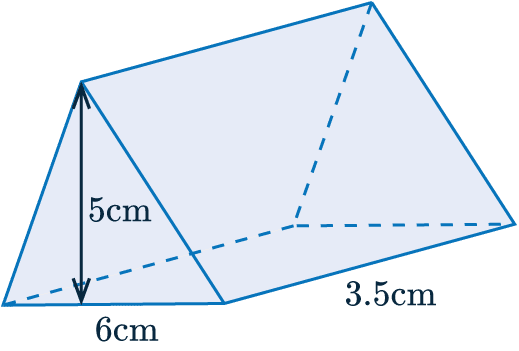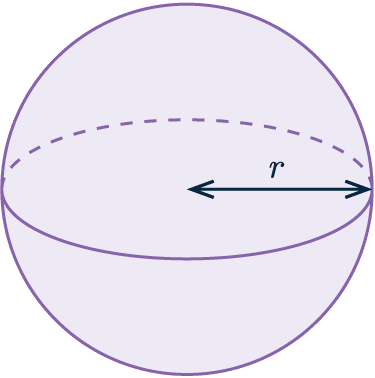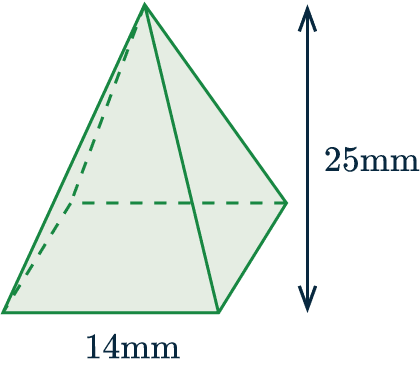# Volume of 3D Shapes

GCSELevel 6-7Edexcel iGCSE

## Volume of 3D Shapes

The volume of a shape is a measure of how much space it occupies.

## Volume of Prisms

The volume of a prism can be calculated as follows:

$\text{Volume of prism}=\textbf{\textcolor{red}{area of cross section}} \times \textbf{\textcolor{blue}{length}}$

This formula can be used for any form of prism, this includes, but is not limited to, cubes, cuboids and cylinders.

$\text{Volume of a Cuboid}=\textbf{\textcolor{red}{Width}} \times \textbf{\textcolor{red}{Height}} \times \textbf{\textcolor{blue}{Length}}$

This can be simplified for a cube since all three measurements are equal:

$\text{Volume of a Cube}=\textbf{\textcolor{red}{Width}}\textcolor{red}{\boldsymbol{^3}}$

$\text{Volume of a Cylinder}=\textcolor{red}{\boldsymbol{\pi r^2}} \times \textbf{\textcolor{blue}{Length}}$

Notice the use of $\boldsymbol{\textcolor{red}{\pi r^2}}$ because the cross section is circular.

This can be expanded to more complex prisms.

Example: The diagram below shows a triangular prism. Work out the volume of the shape.Firstly, find the cross section. As this is a triangular prism, this just entails finding the area of a triangle.

$\text{Area of Cross Section}=\textcolor{red}{\frac{1}{2} \times 5 \times 6 =15} \text{ cm}^2$

Thus,

$\text{Volume of the prism }=\textcolor{red}{15}\times \textcolor{blue}{3.5}=52.5\text{ cm}^3$ ($3$ sf)

Level 4-5GCSEEdexcel iGCSE

## Volume of a Sphere

The formula for the volume of a sphere, which can be found on the formula sheet, is as follows:

$\text{Volume of a Sphere}=\frac{4}{3}\pi r^3$

Example: The diagram below shows a sphere with a radius of $5 \text{ cm}$Work out the volume of the sphere.

Give your answer to $4$ significant figures.

We are given that the radius is $5 \text{cm}$, we simply need to substitute this into the formula.

$\text{Volume of a sphere} = \frac{4}{3} \pi (5)^3 \approx 523.6 \text{ cm}^3$ ($3$ sf)

Level 6-7GCSEEdexcel iGCSE

## Volume of Cones and Pyramids

The formula for the volume of a cone, which can be found on the formula sheet, is as follows:

$\text{Volume of cone }=\frac{1}{3} \times \textcolor{red}{\pi r^2} \times \textcolor{blue}{\text{ perpendicular height}}$

The formula for the volume of a pyramid, which is not on the formula sheet, is as follows:

$\text{Volume of pyramid }=\frac{1}{3}\times\textcolor{red}{\text{ area of base }}\times\textcolor{blue}{\text{ perpendicular height}}$

Example: The diagram below shows a square-based pyramid.Determine the volume of the pyramid.

In this instance, the base is square. So the area of the base is simply:

$\textcolor{red}{14} \times \textcolor{red}{14} = \textcolor{red}{196} \text{ mm}^2$

So,

$\text{Volume of pyramid }=\frac{1}{3}\times\textcolor{red}{196}\times\textcolor{blue}{25} \approx 1633.33 \text{ mm}^3$

Level 6-7GCSEEdexcel iGCSE

## Volume of 3D Shapes Example Questions

Firstly, we need to calculate the cross sectional area.

The cross section is a trapezium, so $\frac{a+b}{2}h$ must be used.

$\frac{45+60}{2} \times 20 =1050 \text{ cm}^2$

Next, we must multiply by the length of the prism.

$1050 \times 80 = 84000 \text{ cm}^3$

The volume for a square-based pyramid is calculated using the following formula:

$\text{Volume }=\frac{1}{3} \times \text{base} \times \text{height}$

The base area is simply a square, so:

$5\times5=25 \text{ cm}^2$

The volume is:

$\text{Volume }=\frac{1}{3} \times 25 \times 12=100\text{ m}^3$

The formula for the volume of a pyramid is:

$\text{Volume }=\frac{1}{3} \times \text{base} \times \text{height}$

This results in:

$\frac{1}{3}\times 18 \times (x+5)=6(x+5)$

Setting this equal to the known volume results in a linear equation:

$6(x+5)=54$

Which can be solved as follows:

$6(x+5)\div6=54\div6$

$x+5=9$

$x=9-5=4\text{ cm}$

To begin with, simply imagine this as two separate shapes. First we will find the volume of the cylinder using:

$\text{Volume of a Cylinder} = \pi r^2 \times \text{ height}$

This results in:

$\pi \times 2.3^2 \times 5.6= 29.624 \pi \text{ cm}^3$

This has been left in terms of $\pi$ for the sake of accuracy.

Next calculate the volume of the hemisphere, which is simply half a sphere:

$\text{Volume of a Hemisphere}= \frac{1}{2} \times \frac{4}{3} \pi r^3$

Resulting in:

$\frac{1}{2} \times \frac{4}{3} \pi \times 2.3^3=8.111\pi \text{ cm}^3$

Finally, add the two volumes together:

$8.111\pi + 29.624 \pi =119 \text{ cm}^3$ (sf)

## You May Also Like...### MME Learning Portal

Online exams, practice questions and revision videos for every GCSE level 9-1 topic! No fees, no trial period, just totally free access to the UK’s best GCSE maths revision platform.

£0.00